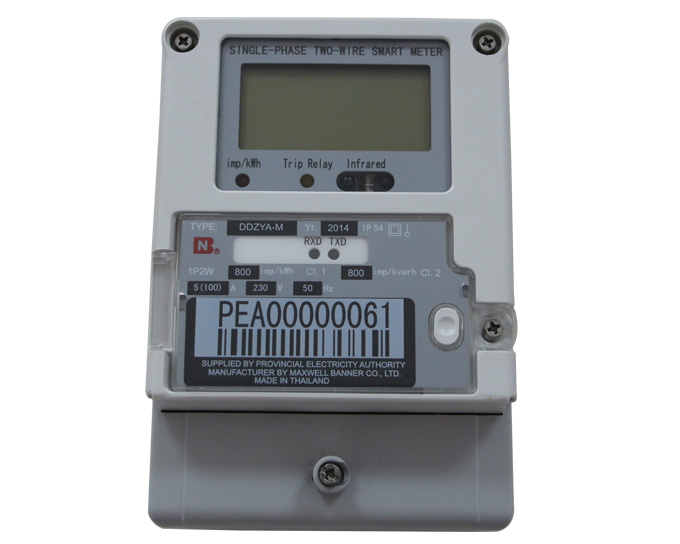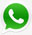News

Home > News

# How much you know electronic energy meter?

Apr 26, 2017

Electronic energy meters can further be classified as electronic analog energy meter and digital energy meter.In analog type electronic energy meters, voltage and current values are obtained by voltage dividers and current transformers. An ‘analog to digital converter’ converts these analog values into digitized samples. These samples are then converted into corresponding frequency signals by a frequency converter. These frequency pulses are then fed to a counter which displays the amount of used energy.

A digital energy meter displays the readings of energy used on a digital display (LCD or LED). No moving parts are present in this type of energy meters. Thus, these are also called as ‘static energy meters’. A digital meter consists of instrument transformers (to sample current and voltage), analog to digital converters, microcontroller etc. The input voltage/current is compared to with a programmed reference voltage and current and then the data gets converted into digital form. The digital data is the processed with appropriate operations in a microcontroller which is then displayed on an LCD or LED display.

••